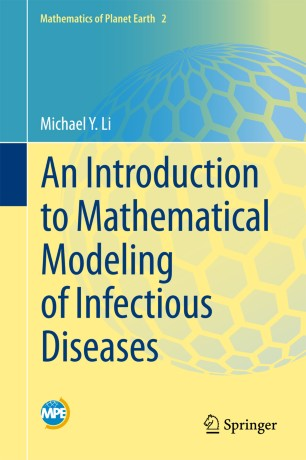# An Introduction to Mathematical Modeling of Infectious Diseases

• Michael Y. LiTextbook

Part of the Mathematics of Planet Earth book series (MPE, volume 2)

1. Front Matter
Pages i-x
2. Michael Y. Li
Pages 1-33
3. Michael Y. Li
Pages 35-77
4. Michael Y. Li
Pages 79-101
5. Michael Y. Li
Pages 103-124
6. Michael Y. Li
Pages 125-150
7. Back Matter
Pages 151-156

### Introduction

This text provides essential modeling skills and methodology for the study of infectious diseases through a one-semester modeling course or directed individual studies.  The book includes mathematical descriptions of epidemiological concepts, and uses classic epidemic models to introduce different mathematical methods in model analysis.  Matlab codes are also included for numerical implementations.

It is primarily written for upper undergraduate and beginning graduate students in mathematical sciences who have an interest in mathematical modeling of infectious diseases.  Although written in a rigorous mathematical manner, the style is not unfriendly to non-mathematicians.

### Keywords

Mathematical Epidemiology Infectious Disease Mathematical Modelling Public Health Kermack-McKendrick Model Nonlinear Least Squares Viral Dynamics Differential Equations Stability Analysis Bifurcation Basic Reproduction Number Parameter Estimation Mathematics of Planet Earth

#### Authors and affiliations

• Michael Y. Li
• 1
1. 1.Mathematical and Statistical SciencesUniversity of AlbertaEdmontonCanada